Software DevelopmentLearn How Data Types And Operators Are Used In Scala

# Learn How Data Types And Operators Are Used In ScalaThe data types available in Scala are similar to those available in Java and other modern programming languages. To use data types effectively in Scala there are four key concepts that need to be understood. These are literals, values, variables and types. This tutorial assumes you have a Scala development environment set up in Eclipse or your favorite IDE. If you have not please refer to setting up a Scala development environment in eclipse tutorial. Each concept will be briefly explained. A literal refers to data that is placed directly in code. For example look at the code shown below.

```object HelloWorld {
def main(args: Array[String]): Unit = {
println("Hello, world!")
}
}```

This is the well known hello world program that prints “Hello World” on the screen. In this code “Hello world!” is a literal. Start eclipse and create a new Scala project, add a HelloWorld object and add the code above. Save and run it as a Scala program.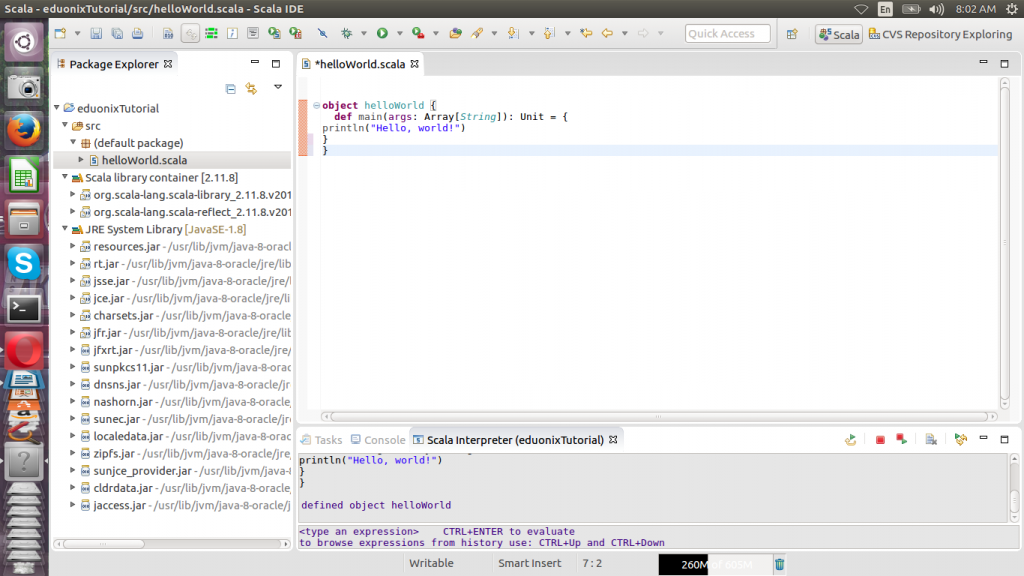Beginning with Scala 2.10.0 you are able to create literals by directly referencing variables. Such a mechanism is referred to as string interpolation and literals created this way are called processed literals. There are three interpolation methods available in Scala. These are s, f and raw. To process a string you just add any of the three methods before the string.

The s method allows you to reference a variable and add it to the string. For example if we expand the hello world program and create a value then we can reference it in the string literal. The expanded program is shown below.

```object HelloWorld {
def main(args: Array[String]): Unit = {
val tutor = “Eduonix learning”
println(s"Hello world!, \$tutor" )
}
}```

The output from our revised program will be Hello world! Eduonix learning.

The f method is used to format strings using a specified style. For example expanding on previous example we can create a number and display it using no decimals.

```def main(args: Array[String]): Unit = {
val tutor = “Eduonix learning”
val daysYear = 365
println(s"Hello world!, \$tutor, \$daysYears%0.f" )
}```

A unit of storage that is immutable is referred to as a value. When the unit of storage is defined it is possible to assign data but it cannot be reassigned. Simply put it cannot be changed once it has been defined. The keyword val is used to define a value. To demonstrate an example start Scala in interactive mode by running scala at a terminal. Below are examples of defining values.

```val name = “sammy”
val age =78
val weight = 34.8```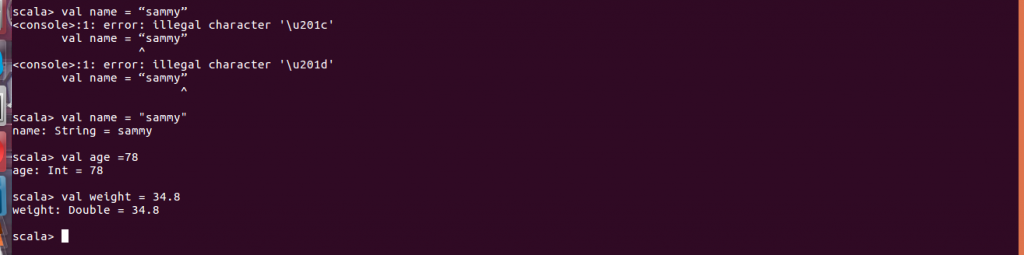A unit of storage that is mutable is referred to as a variable. When this unit of storage is defined you assign data which you can reassign later. In simple terms a definition can be changed. The keyword var is used to define a variable. Below are examples of how to define variables

```var tutor = “eduonix”
var courseSeries = 1
var durationHours = 2.5```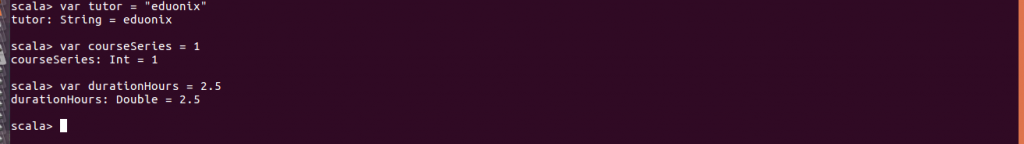The various kinds of data available in Scala are referred to as data types. Everything in Scala is an object and there are no primitive types like those found in Java. The types available in Scala are discussed below.

Byte is used to store signed values that range from -128 to 127. short is used to store signed values that are in the range of -32768 to 32767. Int is used to store values in the range of -9223372036854775808 to 9223372036854775807. Single precision floating point storage is provided by Float type. Storage of double quoted characters is provided by String type. True and false storage is availed by Boolean type. These are some of commonly used types in Scala.
From output when creating values and variables above observe Scala was able to know the data type. This is referred to as type inference. Specifying the data type is still a valid way of defining variables. To demonstrate that let us repeat variable definition by specifying types. Using this approach you specify the data type after the variable name.

```var tutor:String = “eduonix”
var courseSeries:Int = 1
var durationHours:Float = 2.5```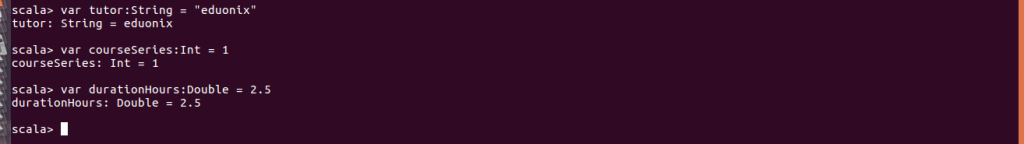Variable scoping exists at three levels depending on where definition happens. Variables defined at an object level are referred to as fields and can be accessed by all methods in the object. It is possible for fields to be accessed outside of an object if access modifiers defining it allow. Fields are allowed to be mutable or immutable. Variables defined within a method have a local scope so they are only accessible within the method. They can be defined as mutable or immutable. Method parameters is the last scope type.

Operators in Scala are categorized into assignment, arithmetic, relational, bitwise and logical. We begin by looking at assignment operators. Arithmetic operators available in Scala are addition (+), substraction (-), multiplication (*), division (+), modulus (%). Below are examples of their use in Scala shell.

```val weight = 45.9
val height =  190
val total = weight + height
val product = weight * height
val divide = weight / height```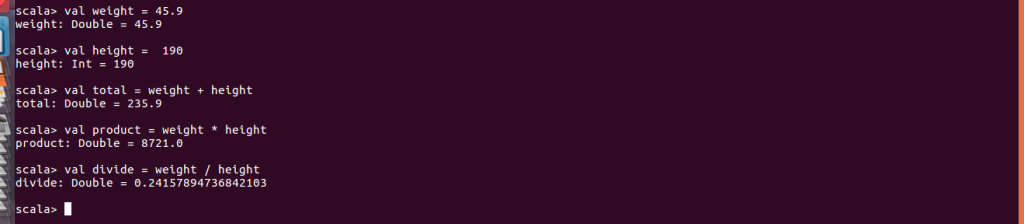Relational operators available are equal to (==), not equal to (!=), greater than (>), less than (<), greater than or equal to (>=) and less than or equal to. The results of their operations are stored in a boolean data type. Examples of their use are shown below

```weight == height
weight > height
weight != height```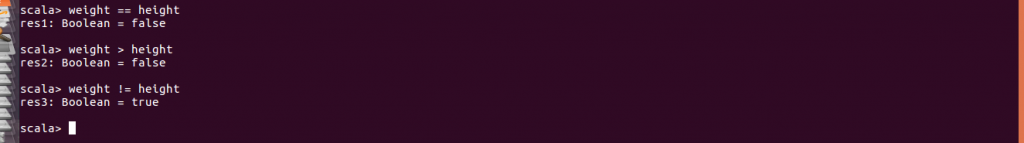Logical operators available AND (&&), OR (||) and NOT (!). The results of such operations are stored in a Boolean type. Use cases are shown below. In the previous example we created weight and height variable. If we have a requirement that both values be greater than a certain value we use &&.To check one of the values is greater than a certain value we use ||. To check weight is not a specific value we use !.

```val wt = weight > 47
val ht = height > 120
wt && height
wt || height```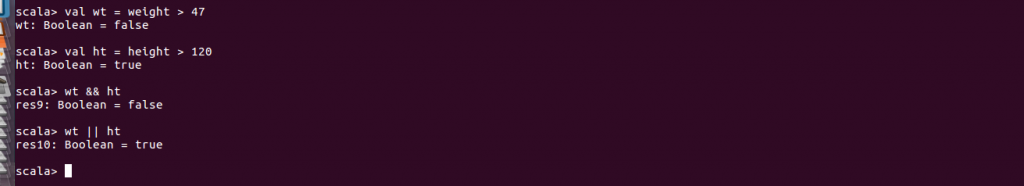The = operator is used to assign the value on the right of the operator to the left. It is possible to combine = and arithmetic operators to combine arithmetic operation and assignment. Examples are shown below.

```val score1 = 89
val score2 =89
score2 += score1 //this is same as score2 = score1 + score2```

This tutorial introduced you to the concepts required to effectively use data types in Scala. Variable definition, mutability and scope were defined. The types of operators available were also discussed.

### Why Is Design Thinking Important In Digital Transformation?

21,501Fans
4,210Followers
104,000Subscribers

### Why Is Design Thinking Important In Digital Transformation?

- Advertisement -